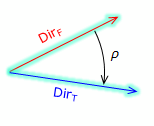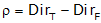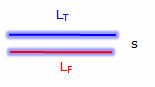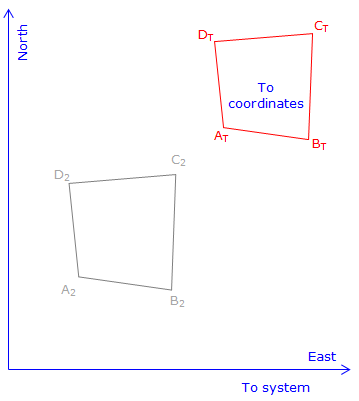## 2. Coordinate Transformation

A coordinate transformation is used to change point positions from one coordinate system to another. Figure H-5 illustrates the same two sets of points from two different coordinate systems:Figure H-5 Two Points Known in Both Systems

The To system is final coordinate system. Points AT and BT are points correctly located in the To system. Points AF and BF are the same points but in a different coordinate system (From system). When plotted in the To system using their From coordinates they appear in a different location. Points CF and DF are also in the From system; we want their coordinates in the To system.

A transformation consists of three elements

• Rotation
• Scaling
• Translation

### a. Rotation

The From coordinates must be rotated to make directions coincide with those of the To system. To determine the rotation angle we need the direction of a common line in both systems.Figure H-6 Direction of Common Line in Both Systems

The difference in directions is the rotation angle, ρ, Figure H-7.Figure H-7 Rotation Angle

Rotation is computed from the direction difference of the same line in both systems. If using two control points, inversing between them in both systems will provide the requisite directions. From the directions the rotation is computed from:Equation H-1

The rotation would be applied to the From coordinates creating a new set, subscripted 1 in Figure H-8:Figure H-8 Rotate From System

### b. Scaling

Scaling is used to increase or decrease distances between the points in order to make them fit in the new system. The distances may be off due to:

• random errors
• systematic errors
• ground to grid distortion (in larger regional systems)
• combinations of the above.

To scale the transformation, we need the length of the same line in both systems, Figure H-9. The length can be from direct measurement (and subsequent adjustment) or by inversing between the coordinates of two common points.Figure H-9 Length of Common Line in Both Systems

Scale is the ratio between the two lengths of the same line in both systems.Figure H-10 Scale

Scale is computed from:Equation H-2

Scaling isn't always necessary depending on the circumstances and allowable error. For example, if a maximum error of 1/5000 is acceptable, then a scale in the range of 1±1/5000 (0.9998 to 1.0002) can be ignored.

When accounting for scale there are three approaches:

1. Unitary - Maintains a 1:1 relationship between the two coordinate systems.

2. Uniform - Enforces a uniform scale (other than 1) in all directions. Only a single scale is computed for the entire transformation.

Both Unitary and Uniform approaches are referred to as a Conformal Transformation because true shapes are maintained.

3. Differential - Scale in the N/S (Y) direction differs from scale in the E/W (X) direction. Two different scales (SN and SE) are computed for the transformation. This is referred to as an Affine Transformation.

Once scale is applied another set of intermediate coordinates, subscripted 2, are created, Figure H-11:Figure H-11 Scaled From System

### c. Translation

The final element are two translations: shift positions in the N/S (Y) and E/W (X) directions. These are determined using coordinates from the previous step with the final coordinates of those points.Figure H-12 Common Point Displacement Between Systems

These two final elements complete the transformation:Figure H-13 Translated From system

Translations are computed from a control point known in both systems (From: NF & EF; To: NT & ET) along with the rotation angle and scale previously determined.Equation H-3

A second control point, if available, can be used for a math check for the translation parameters.

### d. Number of parameters

A coordinate transformation is sometimes referred to as either a three-, four- or five-parameter transformation, Table H-1. The difference between the number of parameters is how scale is applied. Either way, a certain amount of data (control) is needed in both systems.

 Table H-1 Transformation type Three-parameter Four-parameter Five-parameter Parameters TN TE ρ TN TE ρ S TN TE ρ SN SE Control needed in both systems One point's coordinates, one line's directions One point's coordinates, one line's directions, one line's distances One point's coordinates, one line's directions two lines' distances or or or two points two points two points and one line's directions or three points

These are generally the minimal amounts of control needed to uniquely determine the transformation parameters. Any error in the control will be undiscovered and become part of the parameters affecting all points transformed.

Additional control data can be used for math checks. Due to the presence of random errors, different combinations of control will result in slightly different transformation parameters. To get a better model which propagates the error into the final positions, all control should be incorporated in a least squares transformation. Creating such a model manually is time consuming so we will not address least squares transformations here. Many surveying software packages usually include a least squares transformation as an option.

We will examine the four-parameter model as it works well for most ground-based survey applications. Including a uniform scale absorbs smaller random errors satisfactorily as long as good control is used.

### e. Transformation Equations

Once the parameters are determined, they are assembled to create the transformation equations which are used to move other points to the To system.Equation H-4 NT, ET are To system coordinates NF, EF are From system coordinates TE, TN are the translations in East and North directions ρ is the rotation angle S is the scale